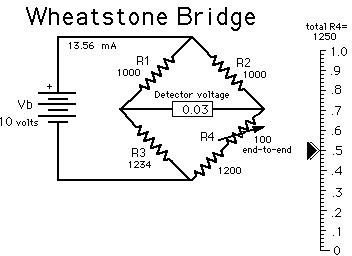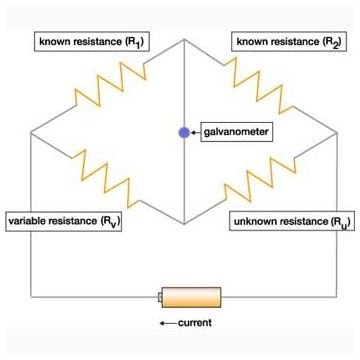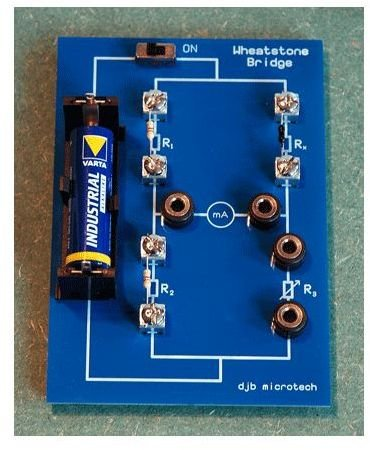# How does a Wheatstone Bridge work ?

## Introduction

Wheatstone bridge is a device which is used to measure the electrical resistance by comparison method. Basically an electrical circuit, wheatstone bridge is used to measure resistance of an unknown resistor by passing current through it. Do you know how does a Wheatstone Bridge work? If not, just find out.

## Brief History

Wheatstone bridge was first founded by Samuel Hunter Christie in the year 1833. However, at that time the inventor couldn’t make out the real use of it. Several years later, Sir Charles Wheatstone claimed the various applications of the device and showed its importance to the people. It was after he described the device was it named the Wheatstone bridge.

## Parts of Wheatstone Bridge

Technically, as mentioned earlier wheatstone bridge is a basic electrically circuit. The arrangement also consists of a power source such as battery and a galvanometer that acts as a connection between the two parallel circuits. The two parallel circuits also consist of two resistors each, out of which the resistances of three are know and the fourth one is of the device whose resistance needs to be found out. The arrangement of the circuit is shown in the figure.## Working

The three known resistances of the parallel branches are already known. The current is allowed to pass through the circuit. When the current passes through the galvanometer, the three resistances are adjusted is such a manner that the galvanometer reading shows zero. The same process can also be carried out by varying the resistance of only one the resistor. Let’s see how.

Now suppose there are 4 resistors R1, R2, R3 and Ru. Ru is the resistor whose resistance is to be found and R2 is the only adjustable resistor. The arrangement is as shown in the figure. R1 and R2 are on one leg and R3 and Rx are on the other leg. Now if is the ratio of resistances of known path, R2/R1 is equal to the unknown path Ru/R3, then the reading at the galvanometer located at the center will show zero. This is done by varying the resistance of R2.

At the point the current in galvanometer is zero; the resistances of all the three known resistors are noted. The resistance of the fourth unknown resistance can be found out by the formula

R2/R1 = Ru/R3

Or Ru = R3. (R2/R1)## Applications

Wheatstone bridge is widely used for measuring small resistances and therefore it is used in applications such as strain gauges and resistance thermometer. Mostly a part of electrical measurement circuits, wheatstone bridge is an integral part of low temperature alarms. For e.g., a thermistor’s resistance is measured by placing the thermistor in place of the unknown resistance in the method described above. The resistance of the thermistor changes as the temperature it is exposed to changes. The temperature and resistance of the thermistor are inversely proportional to each other. This means that if the temperature of the thermistor increases, its resistance decreases. The difference in temperature between the two legs is reflected across the bridge to which an alarm is attached. The temperature difference activates the alarm.Image Credits

www.djb.co.uk/Graphics/Physics/Electrical_Large/Wheatstone.gif

www.opamp-electronics.com/tutorials/images/dc/00179.png

www.frca.co.uk/images/wheatstone_bridge.jpg